Home > AC > Chapter 11 > Lesson 11.1.6 > Problem11-74

11-74.
1. Multiply each expression below using generic rectangles. Homework Help ✎

1. (4x − 1)(3x + 2)

2. (m + 1)(3m − 2)

3. (k − 4)(6 − 5k)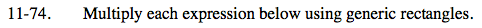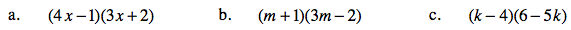Draw the generic rectangle.

Place the two factors on the sides of the generic rectangle as dimensions.

Multiply the dimensions to find the area of each small rectangle.

Add the tile pieces together and simplify by adding like terms.

12x2 + 5x − 2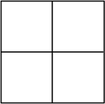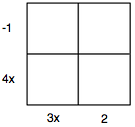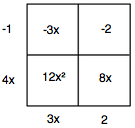3m2 + m − 2

See parts (a) and (b).